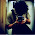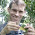## Friday, November 1, 2013

### Random skewers method for comparing covariance matrices

A colleague and friend emailed me the other day to ask me if I had any R code for the so-called "random skewers" matrix comparison method of Cheverud (1996). According to this method, we 'skewer' our target pair of covariance matrices with a set of random selection vectors and then measure the (vector) correlation between the response. The strength of this correlation (compared to the correlation of random vectors) is a measure of the similarity of our matrices. The method is perhaps most clearly described in Cheverud & Marroig (2007).

Well, I don't have code - but it was relatively easy to come up with. The biggest challenge is trying to figure out where the null distribution for the correlation comes from. In Cheverud & Marroig (2007) they suggest that we can just generate random vectors where the elements come from a uniform distribution & then compute the correlations between these random vectors. In my limited trials, though, this seems to result in elevated type I error. Alternatively, perhaps we should generate pairs of random covariance matrices using some model, and then compute the mean correlation between random vectors used to skewer our random covariance matrices. If the model for our covariance matrices is the same one used to generate our null distribution, then we seem to end up with type I error at or around the nominal level; as well as p-values more or less uniform on the interval [0, 1], which is another good sign. Unfortunately, this method is much more computationally intensive.

I've posted code for this function on the phytools page here. It depends on clusterGeneration, so this should be installed before attempting to run the code!

Let's try it using genPositiveDefMat(...,covMehod="unifcorrmat") for our covariance matrix & null hypothesis test:

> source("skewers.R")
> library(clusterGeneration)
> foo<-function(){
X1<-genPositiveDefMat(dim=5,covMethod="unifcorrmat")\$Sigma
X2<-genPositiveDefMat(dim=5,covMethod="unifcorrmat")\$Sigma
skewers(X1,X2,nsim=100,method="unifcorrmat")
}
> X<-replicate(200,foo(),simplify=TRUE)
> mean(unlist(X["p",])<=0.05)
 0.065
> hist(unlist(X["p",]),main="histogram of p",xlab="p", col="grey")

Now let's try it for simulated empirical VCV matrices that are derived from data simulated using the same underlying covariance structure:

> library(mnormt)
> V<-genPositiveDefMat(dim=5,covMethod="unifcorrmat")\$Sigma
> X<-rmnorm(n=30,varcov=V)
> Y<-rmnorm(n=30,varcov=V)
> X<-cov(X)
> Y<-cov(Y)
> skewers(X,Y,method="unifcorrmat")
\$r
 0.9397734
\$p
 0

This is offered without any guarantees. Please let me know if you find any errors.

1.Hi Liam, I'm a phd student at Marroig's lab and we discuss this problem often. We find that generating random matrices for hypothesis testing is quite challenging, because of the difficulty in generating biologically meaningful matrix distributions, especially in regards to integration values. These difficulties can have a big effect in significance. The method you used tends to generate matrices with very low correlations, leading to aligned responses and inflated RS values in the random distribution. Having said that, I agree that using random vectors isn't much of a solution.

The best method we found for generating correlation matrices with a more realistic distribution of high and low correlations can be found here: https://github.com/lem-usp/Morphometrics/blob/master/R/RandomCorr.R and for covariance matrices: https://github.com/lem-usp/Morphometrics/blob/master/R/RandomMatrix.R

In the end, we mostly don't think about the significance all that much, and treat RS as a measure of similarity. Using any method, is the RS in bellow significance, it's usually due to large perceptible differences in matrix structure, and we try to map them using the Selection Response Decomposition method.

thanks!

1.Hi Diogro.

Thanks for the comment. I'm not an expert on this method, so I just tried to do something sensible. The feedback is welcome.

All the best, Liam

Note: due to the very large amount of spam, all comments are now automatically submitted for moderation.